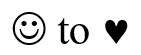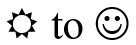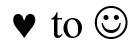Unit 1: Ratio, Rate, & Proportion

# Introduction to Ratios

Ratio is a comparison of one number or quantity with another number or quantity. Ratio shows the relationship between the quantities.

Ratio is pronounced “rā’shō” or it can be pronounced “rā’shēō.” Check out this YouTube video to listen to someone pronouce the word: How to Pronounce Ratio.

You often use ratios, look at these examples:

• Scores in games are ratios. For example, the Penguins won 4 to 3 or the Canucks lost 1 to 5.
• Directions for mixing can be ratios. For example, use 1 egg to each cup of milk or mix 25 parts gas to 1 part oil for the motorcycle.
• Betting odds are given as ratios. For example, Black Jade is a 3 to 1 favourite or the heavyweight contender is only given a 2 to 5 chance to win.
• The scale at the bottom of maps is a ratio. For example, 1 centimetre represents 10 kilometres.
• Prices are often given as ratios. For example, 100 grams for $0.79 or 2 cans for$1.85.

For ratios to have meaning you must know what is being compared and the units that are being used. Read these examples of ratios and the units that are used. A general ratio may say “parts” for the units.

1. It rained four days and was sunny for three days last week. The ratio of rainy days to sunny days was $4:3$. ($4:3$ is properly read “4 is compared to 3” but is often read “4 to 3”).
2. The class has 12 men and 15 women registered. The ratio of men to women in the class is $12:15$.
3. At the barbeque, 36 hot dogs and 18 hamburgers were eaten. The ratio of hot dogs eaten to hamburgers eaten is $36:18$.
4. The class spends 3 hours on English and 2 hours on math each day. The ratio of time spent on English compared to math is $3:2$.

Exercise 1

Write the ratios asked for in these questions using the $:$ symbol (for example, $4:1$). Write the units and what is being compared beside the ratio.

1. Powdered milk is mixed using 1 part of milk powder to 3 parts of water. Write a ratio to compare the milk powder to the water.
Answer: $1:3$ — 1 part of milk powder to 3 parts of water
2. One kilogram of ground beef will make enough hamburger for 5 people. Write a ratio to express the amount of ground beef for hamburgers to the number of people.
3. Seventy-five vehicles were checked by the police. Fifteen vehicles did not meet the safety standards, but 60 of them did. Write a ratio comparing the unsafe vehicles to the safe vehicles.
4. The recipe says to roast a turkey according to its weight. For every kilogram, allow 40 minutes of cooking. Write a ratio comparing time to weight.
5. The 4-litre pail of semi-transparent oil stain should cover 24 square metres of the house siding if the wood is smooth. Write the ratio comparing quantity of stain to the smooth wood surface area.
6. The same 4 L of stain will only cover 16 square metres of the house siding if the wood is rough. Write that ratio.

1. $1:5$        1 kg of beef to 5 people
2. $15:60$   15 unsafe vehicles to 60 safe vehicles
3. $40:1$     40 minutes to 1 kg of turkey
4. $4:24$     4 L of stain to 24 m2 of smooth wood
5. $4:16$     4 L of stain to 16 m2 of rough wood

The numbers that you have been using to write the ratios are called the terms of the ratio.

The order that you use to write the terms is very important. Read a ratio from left to right and the order must match what the numbers mean. For example, 3 scoops of coffee to 12 cups of water must be written $3:12$ as a ratio because you are comparing the quantity of coffee to the amount of water.

If you wish to talk about the amount of water compared to the coffee you have, you would say, “Use 12 cups of water for every 3 scoops of coffee” and the ratio would be written $12:3$.

Ratios can be written 3 different ways:

1. Using the $:$ symbol — $2:5$
2. As a common fraction — $\frac{2}{5}$
• The first number in the ratio is the numerator; the second number is the denominator.
• Ratios written as a common fraction are read as a ratio, not as a fraction. Say “2 to 5,” not “two-fifths.”
3. Using the word “to” — 2 to 5

Exercise 2

Use the ratios you wrote in Exercise 1 to complete the chart.

$:$ Common fraction to
A $1:3$ $\frac{1}{3}$ 1 to 3
B
C
D
E

1. $1:5$ or $\frac{1}{5}$ or 1 to 5
2. $15:60$ or $\frac{15}{60}$ or 15 to 60
3. $40:1$ or $\frac{40}{1}$ or 40 to 1
4. $4:24$ or $\frac{4}{24}$ or 4 to 24
5. $4:16$ or $\frac{4}{16}$ or 4 to 16

Exercise 3

Use the diagram to write a ratio comparing the quantity of each shape, as asked.1.2.3.1. $9:6$ or $\frac{9}{6}$ or 9 to 6
2. $7:9$ or $\frac{7}{9}$ or 7 to 9
3. $6:9$ or $\frac{6}{9}$ or 6 to 9

# Equivalent Ratios

Like equivalent fractions, equivalent ratios are equal in value to each other.

$10:100 = 1:10$

Ratios can be written as common fractions. It is convenient to work with ratios in the common fraction form.

You can then easily:

• Find equivalent ratios in higher terms
• Find equivalent ratios in lower terms
• Find a missing term

Example A

Express $4:5$ in higher terms.

$4:5=\dfrac{4}{5}\longrightarrow \dfrac{4}{5} \times \left(\dfrac{2}{2}\right) \longrightarrow\left(\dfrac{4\times 2}{5\times 2}\right)\longrightarrow\dfrac{8}{10}$

$4:5$ is equivalent to $8:10$

Example B

Express $3:6$ in lower terms.

$3:6=\dfrac{3}{6}\longrightarrow \dfrac{3}{6} \div \left(\dfrac{3}{3}\right) \longrightarrow \left(\dfrac{3\div 3}{6\div 3}\right)\longrightarrow\dfrac{1}{2}$

$3:6$ is equivalent to $1:2$

To find equivalent ratios in higher terms, multiply each term of the ratio by the same number. To find equivalent ratios in lower terms, divide each term of the ratio by the same number.

Exercise 4

Write equivalent ratios in any higher term. You may want to write the ratio as a common fraction first. Ask your instructor to mark this exercise.

1. $5:6= \dfrac{5}{6} \times \left(\dfrac{3}{3}\right)=\left(\dfrac{5\times 3}{6\times 3}\right)=\dfrac{15}{18}=15:18$
2. $4:3$
3. $10:2$
4. $50:1$
5. $9:4$
6. $3:5$

Exercise 5

Write these ratios in lowest terms—that is, simplify the ratios.

1. $4:12=\dfrac{4}{12}\div\left(\dfrac{4}{4}\right)=\left(\dfrac{4\div4}{12\div4}\right)=\dfrac{1}{3}=1:3$
2. $10:5$
3. $7:21$
4. $20:5$
5. $6:14$
6. $2:4$
7. $6:3$
8. $16:8$

Ratios written as a common fraction or using the word “to” will also be correct in this exercise. The terms must be the same.

1. $1:3$
2. $2:1$
3. $1:3$
4. $4:1$
5. $3:7$
6. $1:2$
7. $2:1$
8. $2:1$

Exercise 6

Using a colon, write a ratio in lowest terms for the information given.

1. In the class of 25 students, only 5 are smokers. Write the ratio of smokers to non-smokers in the class. (Note—you must first calculate the number of non-smokers.)
2. The police issued 12 roadside suspensions to drivers out of the 144 who were checked in the road block last Friday. Write the ratio of suspended drivers to the number checked.
3. Twenty-seven students registered for the course and 24 completed it. Write a ratio showing number of completions compared to number enrolled.
4. During an hour (60 minutes) of television viewing last night there were 14 minutes of commercials, so there were only 46 minutes of the actual program! Write the ratio of commercial time to program time.
5. For each pair of coins, write the ratio comparing the value. (Use cents.)
1. A nickel to a dime                    $5:10=1:2$
2. A nickel to a quarter
3. A nickel to a dollar
4. A dime to a nickel
5. A dime to a quarter
6. A dime to a dollar
7. A dollar to a dime

1. $1:4$
2. $1:12$
3. $8:9$
4. $7:23$
1. $1:2$
2. $1:5$
3. $1:20$
4. $2:1$
5. $2:5$
6. $1:10$
7. $10:1$

# Topic A: Self-Test

Mark       /12                  Aim        10/12

1. Write the definitions.
(3 marks)

1. Ratio
2. Terms of the ratio
3. Equivalent ratios

2. Write the ratios asked for in lowest terms. Use the colon style like this: $2:1$. Then write the ratio as it is read, like this: two to one.
(4 marks)

1. The campground had three vacant campsites and 47 occupied sites. Write the ratio of occupied sites to vacant sites. Ratio:                      Read:
2. For every ten dogs in the city, only 2 have current dog licences. Write the ratio of licensed dogs to unlicensed dogs. (Find the number of unlicensed dogs first). Ratio:                      Read:

3. Simplify these ratios.
(5 marks)

1. $9:12$
2. $6:4$
3. $500:1000$
4. $2:9$
5. $35:15$

## Answers to Topic A Self-Test

1. Write the definitions.
1. A ratio is a comparison of one number or quantity with another number or quantity. Ratios show the relationship between the quantities or amounts.
2. Terms of a ratio are the numbers used in the ratio, the parts of the ratio.
3. Equivalent ratios are ratios of equal value to each other.
2. Write ratios in lowest terms.
1. $47:3$
Read: ““47 occupied sites to 3 vacant sites.”
2. $1:4$
3. Simplify the ratios.
1. $3:4$
2. $3:2$
3. $1:2$
4. $2:9$
5. $7:3$